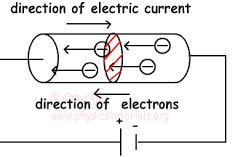Physics

# Define Electric Current

Electric Current: The current is defined as the rate of flow of charges across any cross sectional area of a conductor. If a net charge q passes through any cross section of a conductor in time t, then the current I = q / t, where q is in coulomb and t is in second. The current I is expressed in ampere. If the rate of flow of charge is not uniform, the current varies with time and the instantaneous value of current i is given by,

i = dq/dtCurrent is a scalar quantity. The direction of conventional current is taken as the direction of flow of positive charges or opposite to the direction of flow of electrons.